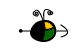Boiling

A liquid boils at a temperature at which its vapor pressure is equal to the pressure of the gas above it. The lower the pressure of a gas above a liquid, the lower the temperature at which the liquid will boil.

The Macroscopic View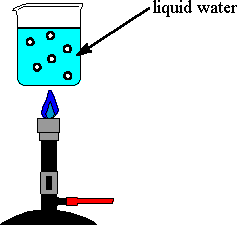• As a liquid is heated, its vapor pressure increases until the vapor pressure equals the pressure of the gas above it.
• Bubbles of vaporized liquid (i.e., gas) form within the bulk liquid and then rise to the surface where they burst and release the gas. (At the boiling temperature the vapor inside a bubble has enough pressure to keep the bubble from collapsing.)
• In order to form vapor, the molecules of the liquid must overcome the forces of attraction between them.
• The temperature of a boiling liquid remains constant, even when more heat is added.

The boiling point of a liquid is the temperature at which its vapor pressure is equal to the pressure of the gas above it.The normal boiling point of a liquid is the temperature at which its vapor pressure is equal to one atmosphere (760 torr).

The Microscopic ViewMicroscopic view inside a bubble in boiling water. The diagram shows the right-hand inner surface of the bubble. Note that water gas and liquid are in equilibrium.

### Factors That Affect the Boiling Point

• Pressure: when the external pressure is:
• less than one atmosphere, the boiling point of the liquid is lower than its normal boiling point.
• equal to one atmosphere, the boiling point of a liquid is called the normal boiling point.
• greater than one atmosphere, the boiling point of the liquid is greater than its normal boiling point.

The following graph shows the boiling point for water as a function of the external pressure. The line on the graph shows the normal boiling point for water.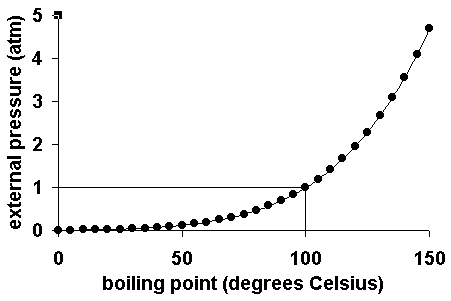• Types of Molecules: the types of molecules that make up a liquid determine its boiling point. If the intermolecular forces between molecules are:
• relatively strong, the boiling point will be relatively high.
• relatively weak, the boiling point will be relatively low.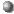= C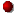= O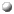= H methyl ether (C2H6O)The normal boiling point of methyl ether is -25oC (i.e., a gas at room temperature). The relatively weak dipole-dipole forces and London dispersion forces between molecules results in a much lower normal boiling point compared to ethyl alcohol. ethyl alcohol (C2H6O)The normal boiling point of ethyl alcohol is 78.5oC (i.e., a liquid at room temperature). Although dipole-dipole forces and London dispersion forces also exist between ethyl alcohol molecules, the strong hydrogen bonding interactions are responsible for the much higher normal boiling point compared to methyl ether.